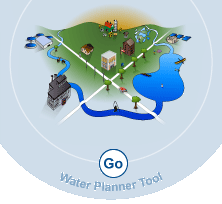Home Introduction Module 1: Water Use and Impacts Module 2: Water Management Risk Assessment Module 3: Case Examples and Links Calculators Reference# Pump Hydraulic Horsepower

The horsepower of a pump can be determined once basic information is known about the pumping station.

Horsepower is the unit of power to define hydraulic or water horsepower. In System International System (SI) it is in kilowatts (kw).

The hydraulic power is the next energy transferred to the water per unit time. The input power delivered by the motor to the pump is called brake horsepower (bHp).

The difference between the brake horsepower and hydraulic power is the pump efficiency.

 Horsepower Calculator (see formula) Head = ft Flow Rate = gpm Specific Gravity = HP = KW = Brake Horsepower Calculator (see formula) HP = HP Pump Efficiency = % Brake Horsepower = Brake KW = SI Calculator Pressure = meters Flow Rate = L/S KW = HP =

## Hydraulic Horsepower and Kilowatt Equations

Hydraulic HP = Head (ft) x Flow Rate (gpm) x (Specific Gravity)
3956
Multiply HP by 0.746 to obtain kilowatts
Hydraulic Kilowatts = (9.81) x Head (meters) x Flow Rate ( L / S ) x (Specific Gravity)
1000
Multiply kilowatts by 1.341 to obtain horsepower

## Brake Horsepower and Motor Efficiency

Brake Horsepower = Hydraulic Power
Pump Efficiency

Once the hydraulic horsepower is known, the brake horsepower can be determined based on the pump efficiency. A pump efficiency of 60% can be used as a conservative estimate for a basic calculation to provide a general approximation.

Many technical references are available that can provide guidance on the use of these equations and the user should refer to these references.

## Sample Horsepower Calculations

Question: Determine the estimated pump horsepower based on the 200 gpm flow rate and 100 ft discharge pressure at the pump.
HP = Head (ft) x Flow Rate (gpm) x (Specific Gravity)
3956
Pump Discharge Pressure = 100 ft
Measured Flow Rate = 200 gpm

## Calculate Horsepower

HP = 100 ft x 200 gpm x 1.0
3956
HP = 5

## Calculate Brake Horsepower

Brake Horsepower = 5
0.6
= 8.3

## SI Units

Pump Discharge Pressure = 30.48 meter
Measured Flow Rate = 12.6 L / S
Kilowatts = 9.81 x 30.48 m x 12.6 L / S x 1.0
1000
Kilowatts = 3.76## Legend

 L/S = Liters per Second gpm = Gallons per Minute HP = Horsepower KW = Kilowatt m/s = Meters per Second m³ = Cubic Meters m² = Square Meters ft² = Square Feet ft³ = Cubic FeetGEMI
1155 Fifteenth Street, NW,
Suite 500
Washington, DC 20005
Phone: 202-296-7449
Fax: 202-296-7442
info@gemi.org